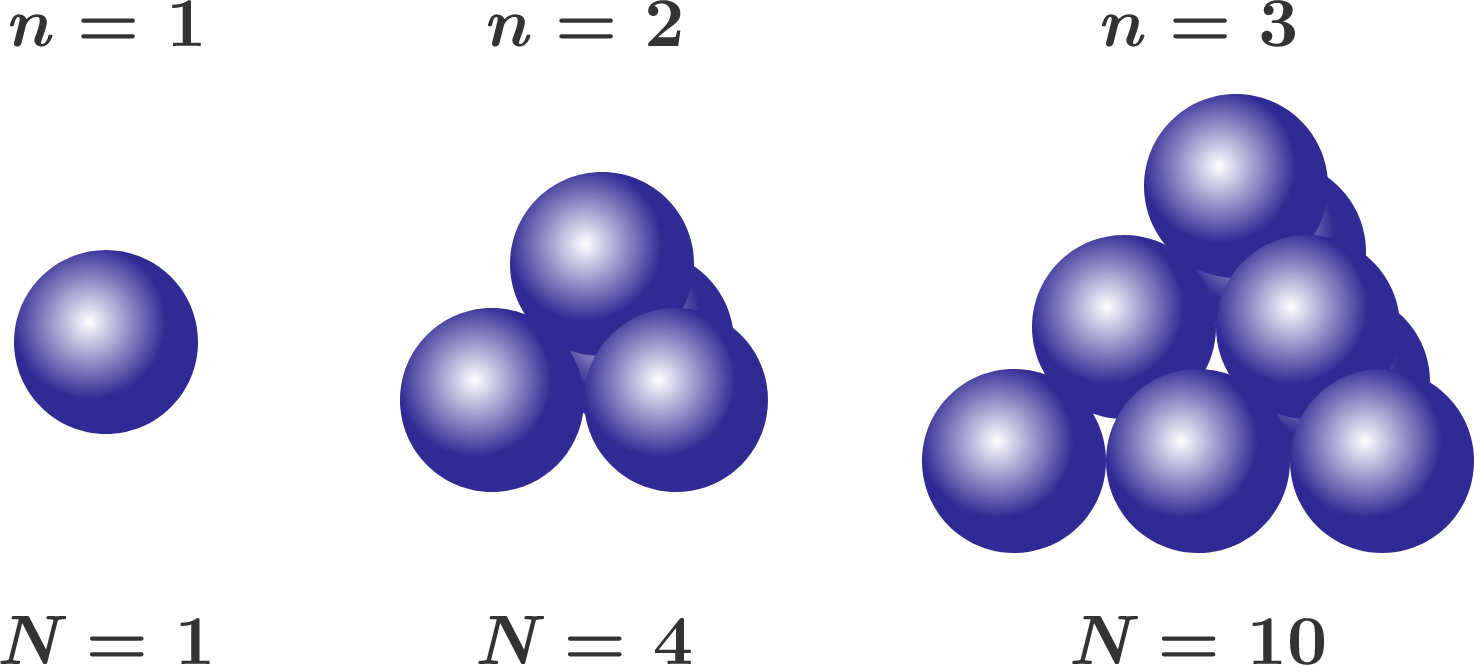# Tennis Ball Pyramid

Algebra Level 2

We build triangle pyramids from $N$ tennis balls, each with number of layers $n=$ 1, 2, 3, or ... (The base of the pyramid is also a triangle.) The trivial pyramid with a single layer consists of a single ball. Two layers require four balls, while the three-layer pyramid requires ten balls.

What is the number $N$ of balls required for the pyramid with $n = 100$ layers?Hint: Find a polynomial $N (n)$ that describes the number of balls as a function of the edge length $n$.

×